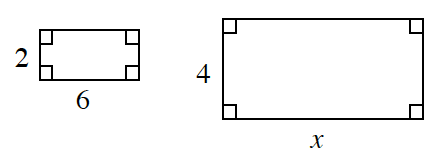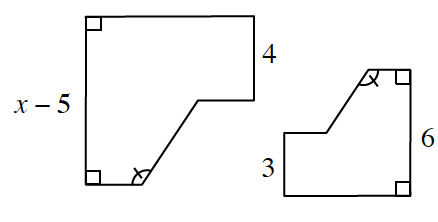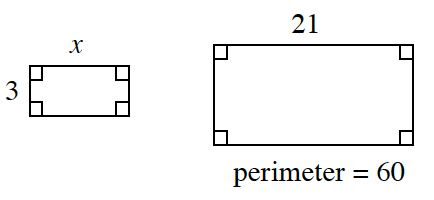### Home > INT2 > Chapter 3 > Lesson 3.1.1 > Problem3-9

3-9.

Each pair of polygons below is similar. Review what you have learned about similarity as you solve for $x$.

1.Ratios of corresponding sides are equal in similar figures.

1.One figure has been rotated, so the $3$ and $4$ are corresponding sides.

1.If the perimeter of the right rectangle is $60$, what is the width?